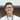# Move Zeroes To End

April 25, 2021

This may or may not be the optimal solution, DO NOT blindly memorize my solutions

From Cassidy’s weekly newsletter.

Target audience: Beginners in js (please feedback!)

# This week’s question:

Given an integer array, move all 0s to the end of it while maintaining the relative order of the non-zeroes. Bonus: do this without making a copy of the array!

## Example:

$moveZeroes([0,2,0,3,8])$ [2,3,8,0,0]

## Filtering zeroes and adding at the end

One of the first solution that comes to mind for this question is to remove the zeroes, and append it to the end of the array.

const moveZeroesNaive = (arr) => {
let noZeroes = arr.filter((num) => num !== 0);
return [...noZeroes, ...new Array(arr.length - noZeroes.length).fill(0)];
};

This solution works, and there are probably other ways to code this such that we wont make another copy of an array. Filter makes a copy, and spreading also copies by value in js.

## Bubbling

const moveZeroesBubble = (arr) => {
for (let i = 0; i < arr.length; i++) {
if (arr[i] === 0) {
for (let j = i; j < arr.length - 1; j++) {
arr[j] = arr[j + 1];
arr[j + 1] = 0;
}
}
}
return arr;
};

const arr = [0, 2, 0, 3, 8];
moveZeroesBubble(arr);
console.log(arr);

This solution is similar to what we expect of bubble sort. Every time we face a zero, we bubble a zero to the end. This has a complexity of O(n^2) and takes no extra space.

## Abusing sort

One thing we can try is to make use of tools already available to us. In js, we have an in-place sort, and can rely on the js engine to have a decent sort algorithm, of O(n log n) complexity (probably timsort)

const moveZeroes = (arr) => arr.sort((a, b) => (b === 0 ? -1 : 0));

const arr = [0, 2, 0, 3, 8];
moveZeroes(arr);
console.log(arr);

What we can do is to not sort according to both values, but put in a comparison result that checks if one of the array is a zero or not! (if we did a === 0 instead, we get the 0s at the front)Ten Zhi Yang is a dev in Singapore. Disagree with me? @ me on twitter. Like my post? @ me on twitter too!